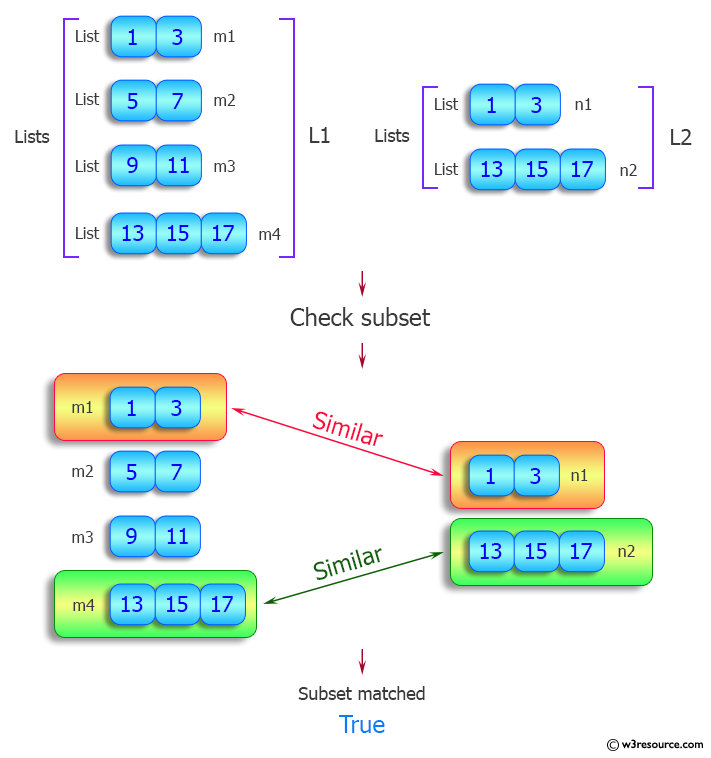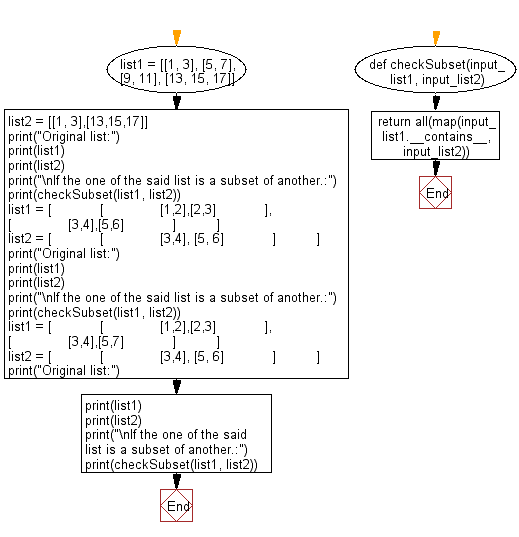﻿ Python: Check if a nested list is a subset of another nested list - w3resource# Python: Check if a nested list is a subset of another nested list

## Python List: Exercise - 92 with Solution

Write a Python program to check if a nested list is a subset of another nested list.

Sample Solution:

Python Code:

``````def checkSubset(input_list1, input_list2):
return all(map(input_list1.__contains__, input_list2))

list1 = [[1, 3], [5, 7], [9, 11], [13, 15, 17]]
list2 = [[1, 3],[13,15,17]]
print("Original list:")
print(list1)
print(list2)
print("\nIf the one of the said list is a subset of another.:")
print(checkSubset(list1, list2))

list1 = [
[
[1,2],[2,3]
],
[
[3,4],[5,6]
]
]
list2 = [
[
[3,4], [5, 6]
]
]
print("Original list:")
print(list1)
print(list2)
print("\nIf the one of the said list is a subset of another.:")
print(checkSubset(list1, list2))
list1 = [
[
[1,2],[2,3]
],
[
[3,4],[5,7]
]
]
list2 = [
[
[3,4], [5, 6]
]
]
print("Original list:")
print(list1)
print(list2)
print("\nIf the one of the said list is a subset of another.:")
print(checkSubset(list1, list2))
```
```

Sample Output:

```Original list:
[[1, 3], [5, 7], [9, 11], [13, 15, 17]]
[[1, 3], [13, 15, 17]]

If the one of the said list is a subset of another.:
True
Original list:
[[[1, 2], [2, 3]], [[3, 4], [5, 6]]]
[[[3, 4], [5, 6]]]

If the one of the said list is a subset of another.:
True
Original list:
[[[1, 2], [2, 3]], [[3, 4], [5, 7]]]
[[[3, 4], [5, 6]]]

If the one of the said list is a subset of another.:
False
```

Pictorial Presentation:Flowchart:## Visualize Python code execution:

The following tool visualize what the computer is doing step-by-step as it executes the said program:

Python Code Editor:

Have another way to solve this solution? Contribute your code (and comments) through Disqus.

What is the difficulty level of this exercise?

Test your Python skills with w3resource's quiz

﻿

## Python: Tips of the Day

```print(2_000_000)
```2000000